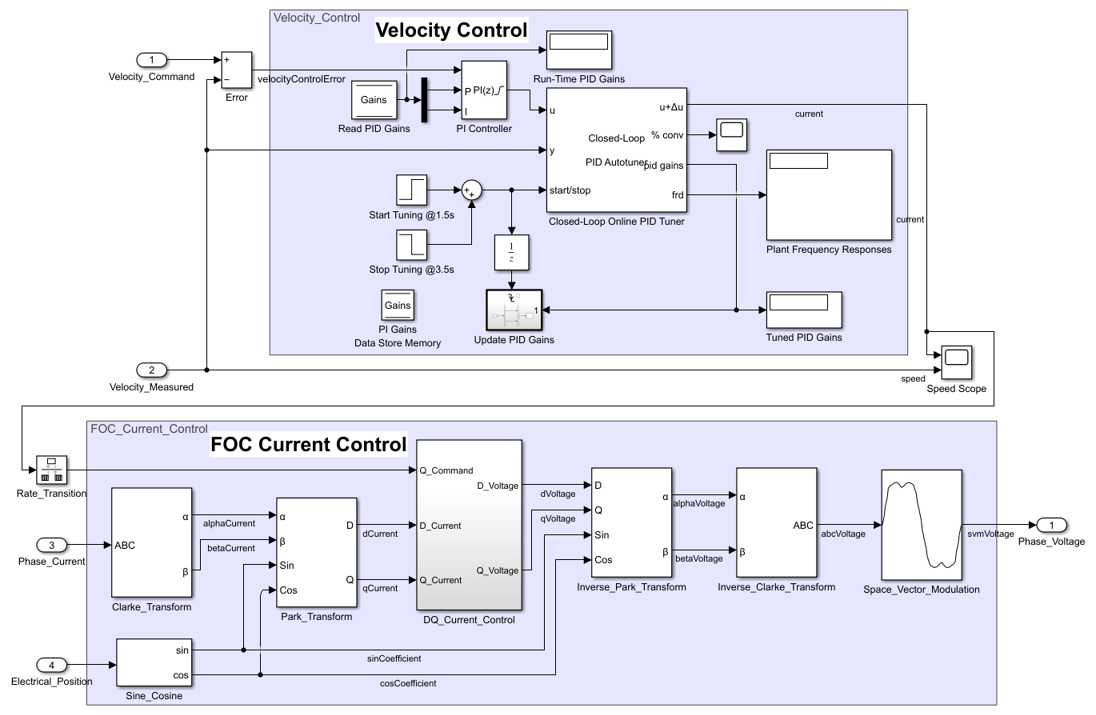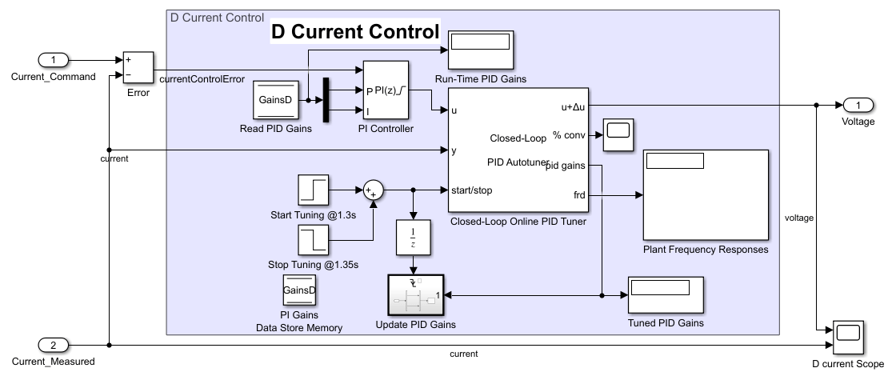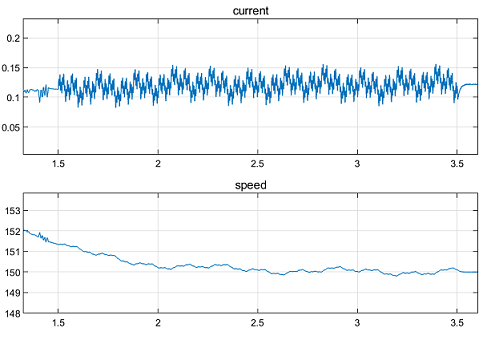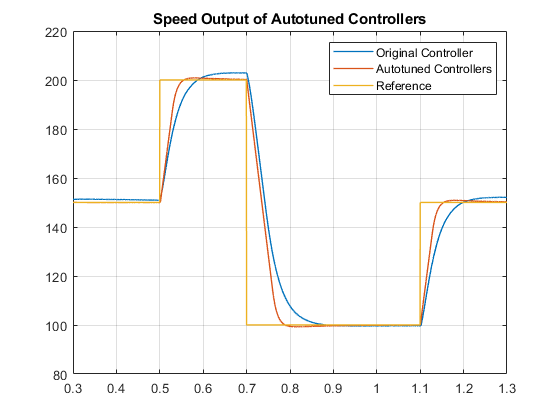Documentation

## Tune Field-Oriented Controllers Using Closed-Loop PID Autotuner Block

This example shows how to use the Closed-Loop PID Autotuner block to tune Field-Oriented Control (FOC) for a permanent magnet synchronous machine (PMSM) in just one simulation.

### Introduction of Field-Oriented Control

In this example, field-oriented control (FOC) for a permanent magnet synchronous machine (PMSM) is modeled in Simulink® using Simscape™ Electrical™ components.

```mdl = 'scdfocmotorPIDTuning'; open_system(mdl) ```Field-oriented control (FOC) controls 3-phase stator currents as a vector. FOC is based on projections, which transform a 3-phase time- and speed-dependent system into a two coordinate time-invariant system. These transformations are the Clarke Transformation, Park Transformation, and their respective inverse transforms. These transformations are implemented as blocks within the Controller_Algorithm subsystem.The advantages of using FOC to control AC motors include:

• Torque and flux controlled directly and separately

• Accurate transient and steady-state management

• Similar performance compared to DC motors

The Controller_Algorithm subsystem contains all three PI controllers. The outer-loop PI controller regulates the speed of the motor. The two inner-loop PI controllers control the d-axis and q-axis currents separately. The command from the outer loop PI controller directly feeds to the q-axis to control torque. The command for the d-axis is zero for PMSM because the rotor flux is fixed with a permanent magnet for this type of AC motor.

The existing speed PI controller has gains of P = 0.08655 and I = 0.1997. The current PI controllers both have gains of P = 1 and I = 200.

The controller gains are stored in a Data Store Memory block and provided externally to each PID block. When the tuning process for a controller is complete, the new tuned gains are written to the Data Store Memory block. This configuration allows you to update your controller gains in real-time during the simulation.

### Closed-Loop PID Autotuner Block

The Closed-Loop PID Autotuner block allows you to tune one PID controller at a time. It injects sinusoidal perturbation signals at the plant input and measures the plant output during a closed-loop experiment. When the experiment stops, the block computes PID gains based on the plant frequency responses estimated at a small number of points near the desired bandwidth. For this FOC PMSM model, the Closed-Loop PID Autotuner block can be used for each of the three PI controllers.

This workflow applies when you have initial controllers that you want to retune using the Closed-Loop PID Autotuner block. The benefits of this approach are:

1. If there is an unexpected disturbance during the experiment, it will be rejected by the existing controller to ensure safe operation.

2. The existing controller will keep the plant running near its nominal operating point by suppressing the perturbation signals.

When using the Closed-Loop PID Autotuner block for both simulations and real-time applications:

• The plant must be either asymptotically stable (all the poles are strictly stable) or integrating. The autotuner block does not work with an unstable plant.

• The feedback loop with the existing controller must be stable.

• To estimate plant frequency responses more accurately in real time, minimize the occurrence of any disturbance in the FOC PMSM model during the experiment. The autotuner block expects the plant output to be the response to the injected perturbation signals only.

• Because the feedback loop is closed during the experiment, the existing controller suppresses the injected perturbation signals as well. The advantage of using closed-loop experiment is that the controller keeps the plant running near the nominal operating point and maintains safe operation. The disadvantage is that it reduces the accuracy of frequency response estimation if your target bandwidth is far away from the current bandwidth.

### Connect Autotuner with Plant and Controller

Insert the Closed-Loop PID Autotuner block between the PID block and the plant for all three PI controllers, as shown in the FOC PMSM model. The `start/stop` signal starts and stops the closed-loop experiment. When no experiment is running, the Closed-Loop PID Autotuner block behaves like a unity gain block, where thesignal directly passes to.

To view the modified outer-loop control structure, open the Controller_Algorithm subsystem.

```controlSubsystem = [mdl '/Controller_Algorithm']; open_system(controlSubsystem) ```View the modified d-axis current controller. The modified q-axis controller has an identical structure.

```open_system([controlSubsystem '/DQ_Current_Control/D_Current_Control']) ```### Configure Autotuner Block

After connecting the Closed-Loop PID Autotuner block with the plant model and PID block, configure the tuning and experiment settings.

On the Tuning tab, there are two main tuning settings:

• Target bandwidth - Determines how fast you want the controller to respond. In this example, choose `5000` rad/sec for current control and `100` rad/sec for speed control.

• Target phase margin - Determines how robust you want the controller to be. In this example, choose `70` degrees for current control and `90` degree for speed control.

On the Experiment tab, there are three main experiment settings:

• Plant Type - Specifies whether the plant is asymptotically stable or integrating. In this example, the FOC PMSM model is stable.

• Plant Sign - Specifies whether the plant has a positive or negative sign. The plant sign is positive if a positive change in the plant input at the nominal operating point results in a positive change in the plant output when the plant reaches a new steady state. Otherwise, the plant sign is negative. If a plant is stable, the plant sign is equivalent to the sign of its dc gain. If a plant is integrating, the plant sign is positive (or negative) if the plant output keeps increasing (or decreasing). In this example, the FOC PMSM model has a positive plant sign.

• Sine Amplitudes - Specifies the amplitudes of the injected sine waves. In this example, choose `0.25` for the current controllers and `0.01` for the speed controller to ensure the plant is properly excited within the saturation limit. If the excitation amplitude is either too large or too small, it will produce inaccurate frequency response estimation results.

Because the Closed-Loop PID Autotuner block only tunes one PI controller at a time, the three controllers must be tuned separately in the FOC PMSM model. Tune the inner-loop controllers first, and then tune the outer-loop controller.

• The d-axis current controller is tuned between 1.3 and 1.35 sec.

• The q-axis current controller is tuned between 1.4 and 1.45 sec.

• The speed controller is tuned between 1.5 and 3.5 sec.

After tuning each PI controller, the controller gains are updated through the Data Store Memory block.

### Simulating Autotuner Block in Normal Mode

In this example, the FOC PMSM model is built in Simulink. All three controllers are tuned in one simulation. In addition, responses are compared between speed responses before and after tuning the controllers.

Simulation of the FOC PMSM model usually takes a few minutes on your computer due to the small sample time of the power electronics controller of the motor.

```sim(mdl) logsout_autotuned = logsout; save('AutotunedSpeed','logsout_autotuned') ```

The following figure shows the overall simulation result.The following figure shows the current and speed responses during tuning, from 1.3 to 3.5 seconds. The change in current is within `0.1` A and the change in motor speed is within `2` rad/sec (about `1`% deviation).The three PI controllers are tuned with new gains.

• The speed PI controller has gains of P = 0.2785 and I = 2.678.

• The d-axis current PI controller has gains of P = 5.135 and I = 8663.

• The q-axis current PI controller has gains of P = 4.59 and I = 8026.

The same velocity commands are applied before and after the autotuning process. Plot the speed responses before and after the controllers are tuned using the Closed-Loop PID Autotuner block. The speed response curves are aligned in time to compare controller performances side-by-side.

```scdfocmotorPIDTuningPlotSpeed ```After tuning the controllers, the speed response of the AC motor has a faster transient response and smaller steady-state error.

```bdclose(mdl) ```